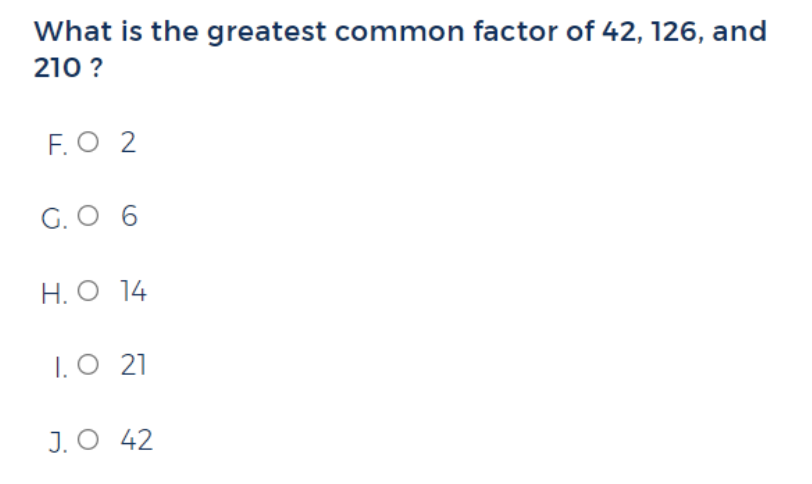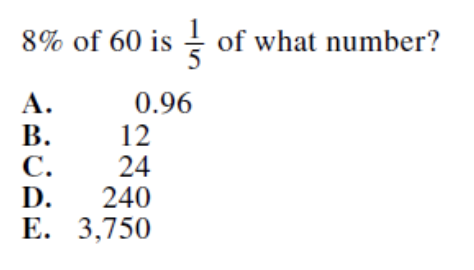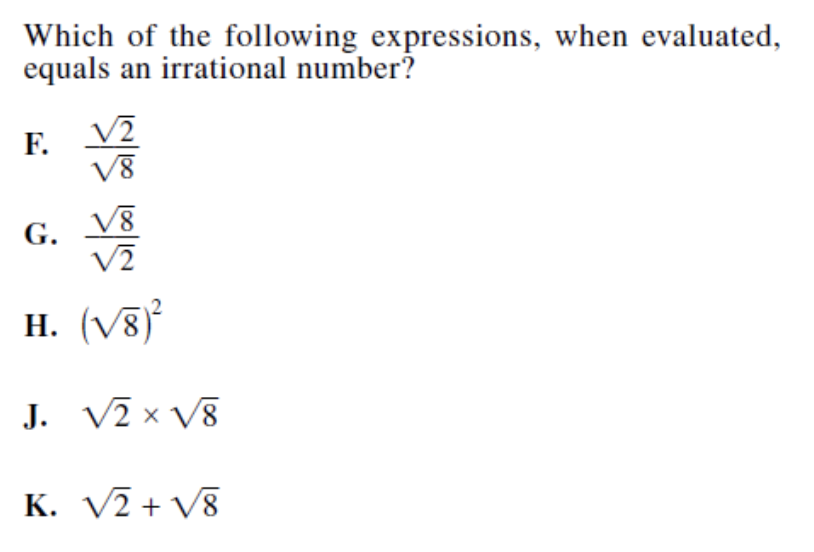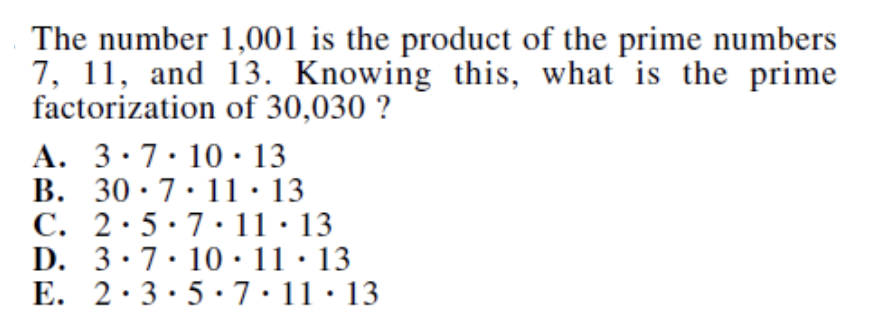### What are your chances of acceptance?

Duke University
UCLA
Unweighted GPA: 3.7
1.0
4.0
SAT: 720 math
200
800
| 800 verbal
200
800

#### Extracurriculars

Low accuracy (4 of 18 factors)Thamira Skandakumar
10

# Guide to ACT Math Number and Quantity + Practice Questions

### Do you know how to improve your profile for college applications?

See how your profile ranks among thousands of other students using CollegeVine. Calculate your chances at your dream schools and learn what areas you need to improve right now — it only takes 3 minutes and it's 100% free.

## What’s Covered:

The manipulation of different types of numbers can be difficult, especially if you haven’t familiarized yourself with the various number types. The ACT Math Test will test your ability to maneuver around these types of questions.

In this post, we’ll cover the number and quantity portion of the ACT Math Test and teach you strategies to answer these questions with ease!

## Overview of ACT Math Number and Quantity

The ACT Math Test consists of 60 multiple-choice questions to be answered within 60 minutes. The test assesses math skills that students typically learn up until the beginning of their senior year of high school. A calculator is allowed for the math portion of the ACT, however, all questions are able to be solved without the use of one.

Number and quantity questions comprise 7-10% of the math test and tests students on their ability to deal with real and complex number systems. Furthermore, students are tested on their ability to manipulate integers, rational exponents, vectors and matrices.

Not only will you be given an overall score for the ACT Math Test, but you will also receive subscores for each of the subsections in the Math test. The ACT also provides the following directions for the math section of the exam which we recommend you become familiar with prior to taking the exam:## How will the ACT Impact My College Chances?

Your ACT scores, just like SAT scores, will be used by most colleges to determine your academic competitiveness. This means that scoring well on the ACT is important, especially if you want a better chance of being accepted into some of the top colleges across the country.

If you want to know how your ACT scores, along with your GPA, high school classes, and extracurriculars will impact your odds of admission, check out CollegeVine’s chancing engine! This tool will take into consideration the above data to determine your chances of getting into various schools. Our chancing engine will even offer tips to help you improve your profile!## 10 Difficult Number and Quantity Questions

Below we will work through 10 different number and quantity questions to not only give you insight as to what these questions look like, but also different strategies to combat these problems.

### Question 1This problem is a number and quantity problem since it requires the student to manipulate ratios. When you are given a set of ratios, you need to be able to recognize that the sum of the individual components equates to a factor of the total value.

In this problem, we know that ingredients A, B and C are mixed in the ratio of 2:3:5 by weight. Using this ratio, we can determine that the total weight is equivalent to 2x + 3x + 5x = 10x. We also know that the net weight of the industrial cleaner is 42. Combining both equations to equate to one another (since both define the net weight of the cleaner) we get 10x = 42.

Therefore:

10x = 42

x = 4.2

If the component of B in the industrial cleaner is 3x, we therefore know that 3 × 4.2 = 12.6

### Question 2Number and quantity questions test your ability to find the lowest common multiple and greatest common factor from a set of numbers. So, make sure you know what both are and how to find them!

To give a brief understanding, the greatest common factor is the largest number that can divide evenly into a set of numbers. Conversely, the lowest common multiple is the smallest quantity that is a multiple of a set of numbers.

In this question, we are asked to find the greatest common factor of 42, 126 and 210. We do this by writing out the prime factorization of each number from the set:

42 = 2 × 3 × 7

126 = 2 × 3 × 3 × 7

210 = 2 × 3 × 5 × 7

Once we have found the prime factorization of each number, we take the common prime factors to the lowest power from each number. This would be 2, 3 and 7. Therefore, the greatest common factor is 2 × 3 × 7 = 42.

While 2, 6, 14 and 21 are all divisible into 42, 126 and 210, 42 is the largest number that is divisible into all three numbers. Therefore, while the other choices are compelling, 42 is the correct answer.

### Question 3Working with number and quantity problems requires you to be able to manipulate fractions. In order to add fractions, you need to have a common denominator between the two. We see here that the two denominators are k and (k+5). The simplest way to find a common denominator is to multiply the two denominators together, therefore, we get k(k+5).

After finding the common denominator, the next thing to do is to convert each fraction to an equivalent fraction with the common denominator we found above. We do this by multiplying (k+5) to the first fraction and k to the second:Therefore, the correct answer is (E)

### Question 4This question is hard because, while it may be easy to solve a matrix equation or find its determinant, it is a little bit more difficult to understand the purpose behind a matrix.

From the problems statement, we realize that b1 and b2 are the costs per pound of bok choy at Market 1 and Market 2, respectively. We also know that r1 and r2 are the costs per pound of rice flour at the same markets. The second matrix depicts just this. The first column of the matrix depicts the prices of the bok choy while the second column depicts the prices of the rice flour. Similarly, the first row and second row of the matrix depicts the costs of each item from Market 1 and Market 2, respectively.

We also know that the unit for both the bok choy and rice flour is cost/pound. The second matrix is depicted with the units cost/pound. Since the first matrix is being multiplied, the units of the first matrix must be pound (pound x (cost/pound)) = cost. This means that the product matrix, [p q], is also cost.

When we expand the matrix equation we see that 0.5r1 + 0.5r2 = q. When we analyze this equation, we see that q is equivalent to the cost for a half pound of rice flour at Market 1 and a half pound of rice flour at Market 2.

### Question 5This problem requires you to work through various percentage and fraction problems. The problem statement asks us to find two fractions that are equivalent to one another:

8% of 60 = ⅕ of x

We know that 8% of 60 is 60(0.08) = 4.8

We know that this is also equal to ⅕ of x:

4.8 = x

x = 4.8 × 5 = 24

## Discover your chances at hundreds of schools

Our free chancing engine takes into account your history, background, test scores, and extracurricular activities to show you your real chances of admission—and how to improve them.

### Question 6After looking at the entire problem statement, we can see that we are asked to find the remainder when n, in the equation in = 1, is divided by 4.  We also know that n is a positive integer.

To solve this problem, we must first determine what value of n makes the equation true. Knowing that i2 = -1 and that we are looking for a value of n that makes the equation equal to 1, we can assume that n = 4. This is because of the following deduction:

i2 = -1

(i2)2 = (-1)2

i4 = 1

Since we have deduced that n = 4, we know that when n is divided by 4 we get a remainder of 0, thereby making answer choice F correct.

### Question 7Number and quantity type problems require you to know the definition of irrational and rational numbers. Here we are expected to find the answer that equates to an irrational number which means that the number can NOT be made by dividing two integers (irrational means no ratio).

We see that answer choice F, √2/√8, can also be written as √2/√(4 × 2). When we simplify this expression we get 1/√4 which equates to ½. Since ½ can be written as a ratio of 1 and 2, answer choice F is a rational number and should be eliminated.

We can use the same logic with answer choice G. When we simplify the ratio we see that √8/√2= √(4 × 2)/√2= 2. An integer is a rational number (an integer can be divisible by 1 which is also an integer), we can also eliminate answer choice G.

Answer H can also be eliminated. (√8)2 = 8 is an integer and therefore a rational number.

Answer J is a product of two square roots. Let us simplify the problem to determine whether or not the value is rational. √2 × √8 = √2 × √(4 × 2) = √2 × 2√2 = 2 × 2 = 4. Since 4 is an integer, we can also reason this value to be a rational number. Therefore answer choice J may also be eliminated.

Through the elimination process, we find that answer choice K is most likely the irrational number when the expression is evaluated. We can double check by solving for √2 + √8. We see that this expression is equivalent to √2 + 2√2 which cannot be further simplified, and is therefore, an irrational number.

### Question 8Here, we are asked to manipulate numbers to determine the speed Kirk was traveling at. From the problem statement, we know that \$17 is fined for each mile per hour (mph) over the posted speed limit. If we know that the posted speed limit is 30 mph and that Kirk was fined \$221, we can figure out the solution in two steps.

We first can determine how many miles Kirk was over the speed limit:

221 = 17x

x = 13

Now that we’ve solved for how many miles Kirk was over the speed limit, you may be inclined to pick answer choice F (I know I was when I did this problem for the first time!). But remember, the question statement is asking for the speed Kirk was traveling at! Therefore, Kirk was traveling at 43 mph since the posted speed limit was 30 mph and Kirk was 13 mph (30 + 13 = 43).

### Question 9This question is quite difficult because it requires us to find an answer statement that is true for all integers. Instead of spending lots of time attempting to figure out the solution on our own, we can easily use the process of elimination to determine the correct answer.

Answer choice F suggests that for the sum of m and n to be odd, m must be an odd integer. For this example, let’s assume m to be 3 (an odd integer). To help determine if this answer choice is correct or not, let’s assume that n is an even integer, for example 4. We know that 3 + 4 = 7 which is an odd integer. However, if we assume n to be an odd integer, let’s say 5, we see that 3 + 5 = 8 which is an even integer. Therefore, we know that answer choice F is incorrect because the property of n is just as important as the property of m.

Rather than testing the other answer choices to see if they are correct, we can use what we learned from answer choice F to find the right answer. We saw that when m was an odd integer and n was also an odd integer, the example produced an even number. This is true for multiple odd integer pairings (e.g. 5 + 7 = 12, 9 + 15 = 24, 27 + 31 = 58).

We later saw that when m was an odd integer and n was an even integer, the two values, when summed, equaled an odd integer. If we test out different odd and even pairings e.g. 5 + 4 = 9, 7 + 8 = 15, 177 + 438 =  615, we see that the expression always equates to an odd integer.

Therefore, we can conclude that the sum of integers m and n will always be odd if m is an odd integer and n is an even integer (answer choice K).

### Question 10After immediately looking into this question, it may seem like using a calculator to find the prime factorization is the best option. However, we are given some vital information that will make this problem easier.

Seeing that the prime factorization of 1,001 is given, we can assume that this number is a factor of 30,030. We indeed can see that 30,030 is a product of 1,001 and 30. Furthermore, we are given the prime factorization of 1,0001, so all we need to do is find the prime factorization of 30!

An easy way to find the prime factorization of a value is to find two numbers that multiply to equate to the given value. We know that 30 = 3 × 10. We know that 3 is a prime number and cannot be broken down any further. However, 10 is not a prime number and is a product of 2 and 5. Both 2 and 5 are prime numbers, therefore, the prime factorization of 30 is 2, 3 and 5.

Now that we know the prime factorization of 30 as well as 1,0001, we can determine that the prime factorization of 30,030 (a product of 30 and 1,001) is 2, 3, 5, 7, 11 and 13. Therefore, answer choice E, 30,030 = 2 × 3 × 5 × 7 × 11 × 13 is correct.

## Final Tips and Strategies

### Learn How to Use Your Calculator

Calculators are allowed for the math portion of the test, which means that it is a good idea to familiarize yourself with your calculator. When taking practice exams or studying up on math problems, you should try practicing with the same calculator that you will be using on test day. You might even be able to learn some useful tricks and shortcuts that will save you plenty of time.

While using a calculator is a good idea, you should be wary of over-relying on your calculator. The ACT Math Test is written so that a calculator is not needed for any problem. For this reason, it may be a good idea to use your calculator only while checking your answers or if you come across a hard problem you don’t know how to solve.

Another trick while taking practice tests is to time yourself while trying to answer a  question with and without a calculator. This will help you determine which types of questions may need a calculator and which won’t, thereby maximizing your test-taking time.

### Stuck? Use Logic

If you come across a difficult question, don’t spend too much time trying to solve the problem. If you immediately recognize that the problem is difficult, skip it and come to it later. When you do return to the difficult problem, try to use logic to eliminate answers that are incorrect. You can do this by comparing the answer choices to each other in an attempt to see how they differ. Differences that you notice can provide clues as to what is being asked of the question or whether the answer choice is too absurd. Once you’ve eliminated as many incorrect answers as possible, it is best to make an educated guess from the answer choices remaining. In the Number and Quantity Practice Problems Section, we use the process of elimination in questions 7 and 9, so you can refer to that for extra help!

The ACT Math Test, unlike the SAT, does not give you a set of equations you can use to help answer questions on the exam. Therefore, it is a good idea to become familiarized with basic formulas that will help you complete the exam. Knowing the purpose of a formula as well as when and how it can be used will help decrease the amount of time you spend on a question.

It is crucial that you become familiarized with important formulas before test day so that you are able to practice with them ahead of time. You can visit CollegeVine’s guide on the 31 ACT math formulas you need to know to help you get started on some of the most useful equations you may need for the exam.

Check out some of our other posts as you study for the ACT Math Test:Short Bio
My name is Thamira Skandakumar and I am from Vancouver, Washington. I am a current fourth year at UCLA studying Bioengineering with a minor in Electrical Engineering. In the summer, I will be moving out to San Diego to work at Medtronic in their Ventilator division.